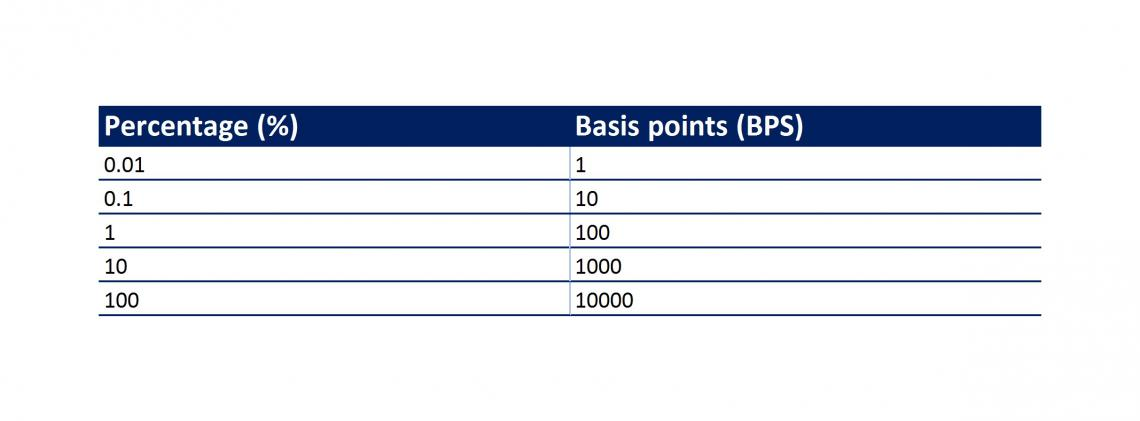# Basis Points (BPS)

What are Basis Points (BPS)?

Basis points (BPS) is a term used in finance for measurement which is equal to 1/100 of a percentage point. It is generally used with respect to securities where the percentage difference is less than 1% but still significant such as interest rates (IR) and debt-related securities.

They are are pronounced as "bips" and abbreviated to "bps", "bp" (singular) and "bips".

The term "basis" comes from the base movement between two percentages, or the spread between two interest rates (IRs).

Examples of use:

• An increase in bond yield from 8.25% to 8.35% is an increase of 10 bps.
• If the Federal Reserve raises rates from 1.65% to 1.85% it is said to be an increase of 20 basis points (bps) of fed rates.
• When the price of an Exchange Traded Fund (ETF) has jumped in price by 2.6% due to favorable event anticipation, it is considered an increase of 260 bps.## Price Value of a Basis Point (PVBP)

The Price Value of a Basis Point (PVBP) measures the absolute value of the change in the price of a bond for a one basis point change in yield. It is another way to measure interest-rate risk, like duration, which measures the percent change in a bond price given a 1% change in rates.

PVBP is a special case of dollar duration. Instead of using a 100 bp (1 percent) change, the price value of a basis point simply uses a 1 basis point (0.01 percent) change. It does not matter if there is an increase or decrease in rates because such a small move in rates will be about the same in either direction. It is also referred to as DV01 or the dollar value change for a 1 bp move.

As an example of PVBP, consider that for every percent change in bond yield, the price of the bond changes by \$20. The dollar duration, in this case, is \$20 and the PVBP is \$0.20 (calculated as \$20/100).

## Basis points (BPS) calculation

The conversion to and from percentage can be easily calculated applying the information below:

• For BPS to percentage, divide by 100.
• For percentage to BPS, multiply by 100.## Using basis points vs percentage

This convention reduces confusion when talking about percentages. For example, consider the following sentence:

"There was an increase of 5 percent on the yield of the bond."

What does this mean? Assuming that the initial yield of the bond was 10%, it is ambiguous whether the bond is now priced at 10.5% or 15%.

What if we had phrased the same sentence as "There was an increase of 50 bps on the yield of the bond." This removes any ambiguity as it communicates that the yield on the bond is 10.5% and not 15%.

This is why basis points are used frequently in areas of finance where communicating in percentages happens to be a common occurrence.

## Basis points (BPS) vs Point in percentage (PIPs)

Pips are commonly used in the foreign exchange markets, where it is the smallest price move that an exchange rate can make based on market convention. Since most currencies are priced to four decimal places and the smallest change happens to be the fourth last decimal.

Hence, a pip is equal to a BP and 1/100 of a percentage. The difference arises in usage, where pips are used in the forex (FX)  markets while basis points are used in the fixed income markets.## Examples of instruments where basis points (BPS) are applied:

BPS are primarily used with respect to yields and interest rates in the context of fixed income assets. However, they may also be used to signify a change in the price of other assets such as equity stocks. Examples of instruments where BPS are applied:### Everything You Need To Master Excel Modeling

To Help You Thrive in the Most Prestigious Jobs on Wall Street.